## TREND INDICATORS

For allowing a trader to determine the market movement direction the trend indicators were worked out. As a rule, they are not restricted by moving averages. They include such instruments as price models, support and resistance lines, trend lines.

As a rule, each indicator has a mathematical formula by means of which it can be calculated.

To the trend indicators the following can be related:

• Average Directional Movement Index — ADX

Technical indicator (Average Directional Movement Index, ADX) helps to determine the price tendency presence. It was worked out and described in the book “New Concepts in Technical Trading Systems” by Welles Wilder.

The simplest trading method on the basis of the directed movement system implies comparison of two indicators of 14-period directivity+DI and 14-period -DI. For this purpose the graph indicators are laid on each other or +DIis deducted from –DI and ticks down lower than -DI.

These simple trading rules are filled up by W.Wilder with the “extreme points rule”. It serves for eliminating false signals and reducing the number of concluded deals. According to the extreme points principle, at the moment of crossing +DI and –DI the “extreme point” should be noticed. If +DI lifts higher than –DI this point is the maximal price for crossing. If +DI moves below –DI this point is the lowest crossing price.

Further the extreme point is used as a market entry level. Thus, after the buy signal (+DI elevated above –DI) it is necessary to wait for the price to exceed the extreme point, and then buy. In case the price cannot overcome the extreme point level then the short position should be kept.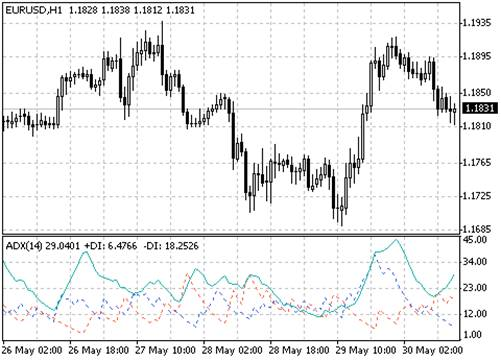Calculation:  ADX = SUM ((+DI - (-DI)) / (+DI + (-DI)), N) / N

Where:
N — the number of periods used for calculation;
SUM (..., N) — amount of N periods;
+DI — the indicator value of positive price movement direction (positive directional index);
-DI — the indicator value of positive price movement direction (negative directional index).

• Bollinger Bands — BB

The Bollinger Bands are similar to Envelopes. The difference between them is in that the bounds of Envelopes are located above and below the moving average curve at a fixed percentage distance, while Bollinger Bands are formed at the distances equal to a certain number of standard deviations. As the value of a standard deviation depends on the volatility the bands regulate their width by themselves: it expands when the market is unstable and reduces in more stable periods.

The Bollinger Bands are usually put on the price chart as well as on the indicator chart. As in the case of Envelopes, the Bollinger Bands interpretation is based on the price feature to stay within the upper and lower bands.

A distinctive feature of Bollinger Bands is their variable area caused by the price volatility. In the periods of significant price changes (i.e. high volatility) the bounds are widening giving the prices a space. In sluggish periods (i.e. low volatility) the bounds are narrowing keeping the prices within the bounds.

Among the indicator peculiarities the following can be distinguished:

1. Sharp price changes which usually occur after band narrowing equal to the volatility lowering.

2. If the prices come out of the bounds then it is needed to wait for the current tendency continuation.

3. If after the peaks and drops out of the bands come the peaks and drops within them the trend turnout is possible.

4. The price movement started from one of the band borders usually reaches the opposite one.

The last inquiry is useful for forecasting the price reference points.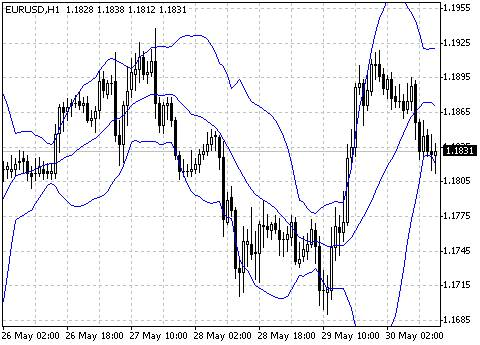Calculation

The Bollinger Bands are formed by three lines. MIDDLE LINE, ML – is a simple moving average.

ML = SUM (CLOSE, N) / N = SMA (CLOSE, N)

TOP LINE, TL is the same middle line removed to the upside by certain number of standard deviations (D).

TL = ML + (D * StdDev)

BOTTOM LINE, BL is the same middle line removed to the downside by certain number of standard deviations.

BL = ML — (D * StdDev)

Where:
SUM (..., N) — amount of N periods;
CLOSE — closing price;
N — the number of periods used for calculation;
SMA — simple moving average;
SQRT — square root;
StdDev — standard deviation:

StdDev = SQRT (SUM ((CLOSE - SMA (CLOSE, N))^2, N)/N)

It is recommended to use 20-period simple moving average as a middle line and 2 simple deviations for calculating the band boundaries. Besides, the moving averages of less than 10 periods are low efficient.

• Moving Average – MA

The technical indicator Moving Average, MA shows an average instrument price for some period of time. During the Moving Average calculation the mathematical averaging of the instrument price for this period is implemented. In proportion to the price changingits average value rises or falls.

There are several types of moving averages (also called arithmetical), exponential, smoothed and weighted. Moving Average can be calculated for any sequential data set, including the opening and closing prices, maximal and minimal price, trading volume and other indicators values. Frequently, the moving averages of the moving averages are used.

The only thing which distinguishes different types of Moving Average from each other – is various weight coefficients which are assigned to the last data. In case of Simple Moving Average all prices of the considered period have the same weight. Exponential Moving Average and Linear Weighted Moving Average make the last prices weightier.

The most wide-spread interpretation method of the price moving average is its dynamics comparison with the price dynamics. When the instrument price moves above the Moving Average a buy signal emerges, and when the price is below the indicator line – sell signal.

This trading system by means of Moving Average is not meant for providing the market entry strictly at its lowest point, and exit – strictly atop. It allows to act corresponding to the current trend: buy just after the prices reach the bottom and sell after the top shaping.

The Moving Averages can be applied to the indicators as well. Amid this, an interpretation of the indicator moving averages is similar to the interpretation of the price moving averages: if the indicator moves above its Moving Average – it means that the upward indicator movement will go on: if the indicator moves below the Moving Average – the downtrend is to be fixed.

Variety of Moving Averages:

• Simple Moving Average (SMA)
• Exponential Moving Average (EMA)
• Smoothed Moving Average (SMMA)

Linear Weighted Moving Average (LWMA)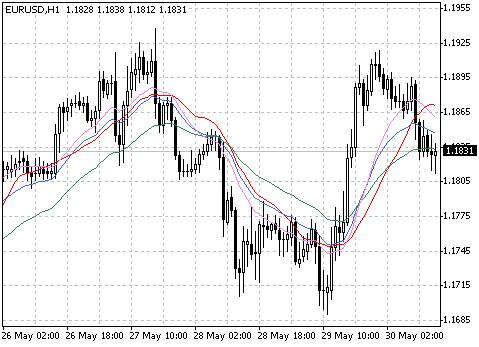Calculation

Simple Moving Average, SMA

Simple and arithmetical moving average is calculated by means of summing up the instrument closing price for a certain number of singular periods (for example 12 hours) with further amount division by the number of periods.

SMA = SUM (CLOSE (i), N) / N

Where:
SUM — amount;
CLOSE (i) — the closing price of the current period;
N — the number of calculation periods.

• Parabolic SAR

The technical indicator Parabolic System SAR was worked out for the trend markets analysis. The indicator is constructed on the price chart. In its own way this indicator is similar to the moving average with the only difference that Parabolic SAR is moving with big acceleration and it can change the position related to price. At the “bullish trend” (Up Trend) the indicator locates below the prices, at the “bearish” one (Down Trend) – above them.

If the price crosses the lines of Parabolic SAR then the indicator turnout takes place, and its next values are placed on the other side from the price. With this indicator “turnout” the starting point will be the highest or lowest price for the previous period. The indicator turnout is a signal of the trend continuation (transit to a correction or flat) or its turnout.

Parabolic SAR perfectly determines the points of market exit. Long positions should be closed when the price decreases below the line of the technical indicator and short – when the price moves above the Parabolic SAR line. Often this indicator is used as a trailing stop line.

If long position is opened (i.e. the price is above the Parabolic SAR) then the indicator line will be moving regardless of the prices direction. The value of the Parabolic SAR line depends on the price movement value.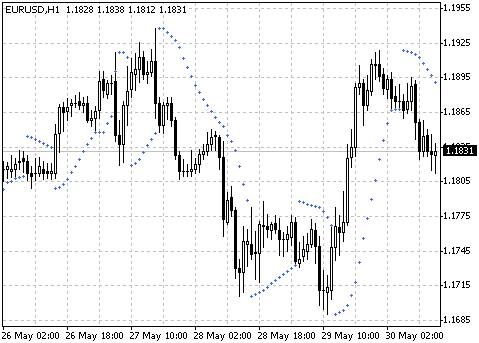Calculation

For long positions:

SAR (i) = ACCELERATION * (HIGH (i - 1) - SAR (i - 1)) + SAR (i - 1)

For short positions:
SAR (i) = ACCELERATION * (LOW (i - 1) - SAR (i - 1)) - SAR (i - 1)

Where:
SAR (i - 1) — value of the Parabolic SAR indicator at the previous bar;
ACCELERATION — acceleration factor;
HIGH (i - 1) — maximal price for the previous period;
LOW (i - 1) – minimal price for the previous period.

The indicator value is advancing if the current bar price is bigger than the preceding one on the bull market and inversely. And the acceleration factor will be doubling that will cause binding of the Parabolic SAR with the price.

• Standard Deviation

The technical indicator (Standard Deviation, StdDev) measures the market volatility. This indicator characterizes the size of price fluctuations in relation to the moving average. Thus, if the indicator value is big then the market is volatile and the bar prices are rather scattered relatively the moving average. If the indicator value is not too big the market has low volatility and the bar prices are quite close to the moving average.

Usually this indicator is used as a component of other indicators. Thus, at calculating the Bollinger Bands the value of a standard instrument deviation is added to its moving average.

The market dynamics consists of interchange of quiet and violent activity periods that is why approach to this indicator is simple:

• If the indicator value is too small, i.e. the market is absolutely quiet then activity surge should be expected soon;

To the contrary, if the indicator is extremely big then this activity is most likely to go downwards.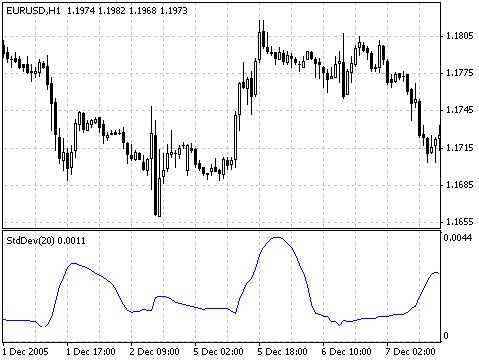Calculation

StdDev (i) = SQRT (AMOUNT (j = i - N, i) / N)

AMOUNT (j = i - N, i) = SUM ((ApPRICE (j) - MA (ApPRICE (i), N, i)) ^ 2)

Where:
StdDev (i) — Standard Deviation of the Current Bar;
SQRT — square root;
AMOUNT(j = i - N, i) — sum of squares from j = i - N to i;
N — smoothing period;
ApPRICE (j) — applied price of j-bar;
MA (ApPRICE (i), N, i) — any moving average of the current bar  for N periods;
ApPRICE (i) — applied price of the current bar.

These indicators are the most widespread. Others are based on them being their derivatives.

Attached Files:

Posted By michaelcapers : 22 September, 2020
##### Related Article
###### Step Kwan averages fl’s – flexible oscillator without redrawing

Step Kwan averages is an oscillator and channel indicator at the same time. It does not redraw, so the signals are rather clear. We recommend you to make a trade when indicator returns to the channel simultaneously with the color change. Nevertheless, you are free to choose your own trade approach.   Step Kwan averages usage example